# Theragun

Grid List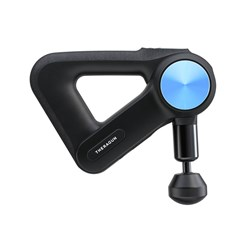Theragun Pro
In Stock
\$599.01
Increase value Decrease value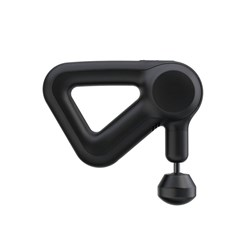Theragun Prime
In Stock
\$329.00
Increase value Decrease value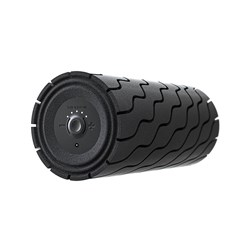\$149.00
Increase value Decrease value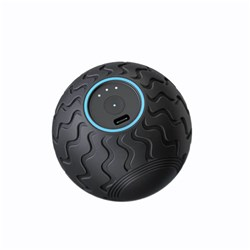\$79.00
Increase value Decrease value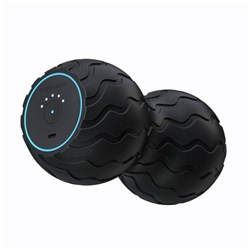\$99.00
Increase value Decrease value
From
Increase value Decrease value
From
Increase value Decrease value
\$89.00
Increase value Decrease value
\$129.00
Increase value Decrease value
\$75.00
Increase value Decrease value
\$29.00
Increase value Decrease value
\$29.00
Increase value Decrease value
\$29.00
Increase value Decrease value
\$79.00
Increase value Decrease value
\$48.99
Increase value Decrease value
\$29.00
Increase value Decrease value
\$29.00
Increase value Decrease value
\$29.00
Increase value Decrease value
\$48.99
Increase value Decrease value
\$48.99
Increase value Decrease value
\$129.00
Increase value Decrease value
\$129.00
Increase value Decrease value
Grid List
##### Products to compare:
Comparing Products